# Triangle angles

The angles α, β, γ in triangle ABC are in the ratio 6:2:6. Calculate size of angles.

Result

α =  77.1 °
β =  25.7 °
γ =  77.1 °

#### Solution:

\alpha = 180 ^\circ \cdot \dfrac{ 6}{ 6+2+6} = 77.1 \text{ ^\circ }
\beta = 180 ^\circ \cdot \dfrac{ 2}{ 6+2+6} = 25.7 \text{ ^\circ }
\gamma = 180 ^\circ \cdot \dfrac{ 6}{ 6+2+6} = 77.1 \text{ ^\circ }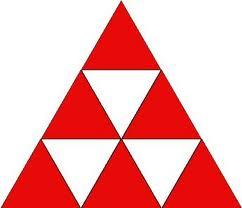Our examples were largely sent or created by pupils and students themselves. Therefore, we would be pleased if you could send us any errors you found, spelling mistakes, or rephasing the example. Thank you!

Leave us a comment of this math problem and its solution (i.e. if it is still somewhat unclear...):Be the first to comment!Tips to related online calculators
Check out our ratio calculator.
Do you have a system of equations and looking for calculator system of linear equations?

## Next similar math problems:

1. A triangleA triangle has an angle that is 63.1 other 2 are in ratio of 2:5 What are the measurements of the two angles?
2. Angles ratioThe internal angles of a triangle are in ratio 1:4:5 What kind of triangle is it? (solve internal angles and write down and discuss)
3. The second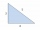The second angle of a triangle is the same size as the first angle. The third angle is 12 degrees larger than the first angle. How large are the angles?
4. AngleDetermine the size of the smallest internal angle of a right triangle which angles forming the successive members of the arithmetic sequence.
5. Right triangle eq2Find the lengths of the sides and the angles in the right triangle. Given area S = 210 and perimeter o = 70.
6. Triangle 42Triangle BCA. Angles A=119° B=(3y+14) C=4y. What is measure of triangle BCA=?
7. In a 2In a thirteen sided polygon, the sum of five angles is 1274°, four of the eight angles remaining are equal and the other four are 18° less than each of the equal angles. Find the angles. .
8. Six-sided polygon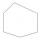In a six-sided polygon. The first two angles are equal, the third angle is twice (the equal angles), two other angles are trice the equal angle, while the last angle is a right angle. Find the value of each angle.
9. Trapezium internal anglesA trapezium where AB is parallel to CD, has angle A : angle D = 4 :5, angle B = 3x-15 and angle C = 4x+20. Find angle A, B, C and D.
10. Three parallelsThe vertices of an equilateral triangle lie on 3 different parallel lines. The middle line is 5 m and 3 m distant from the end lines. Calculate the height of this triangle.
11. Largest angle of the triangleCalculate the largest angle of the triangle whose sides have the sizes: 2a, 3/2a, 3a
12. Three points 2The three points A(3, 8), B(6, 2) and C(10, 2). The point D is such that the line DA is perpendicular to AB and DC is parallel to AB. Calculate the coordinates of D.
13. Inscribed circle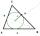Calculate the magnitude of the BAC angle in the triangle ABC if you know that it is 3 times less than the angle BOC, where O is the center of the circle inscribed in the triangle ABC.
14. Angle in RTDetermine the size of the smallest internal angle of a right triangle whose sides constitutes sizes consecutive members of arithmetic progressions.
15. GarageThere are two laths in the garage opposite one another: one 2 meters long and the second 3 meters long. They fall against each other and stay against the opposite walls of the garage and both laths cross 70 cm above the garage floor. How wide is the gara
16. Largest angle of the triangleWhat is the largest angle of the triangle if the second angle is 10° greater than twice the first and the third is 30° smaller than the second?
17. Supplementary angles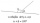One of the supplementary angles is larger by 33° than the second one. Calculate the angles size.